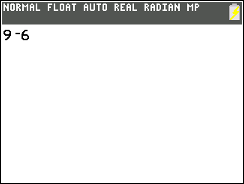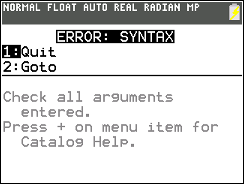# Knowledge Base

## Solution 40403: Correcting Syntax Error when Subtracting or Using Negative Numbers on the TI-83 Plus and TI-84 Plus Family of Graphing Calculators.

### Why do I receive an “ERROR: SYNTAX” or “ERR:SYNTAX” when computing using subtraction or negative numbers on the TI-83 Plus and TI-84 Plus Family of Graphing Calculators?

On the TI-83 Plus and TI-84 Plus family of graphing calculators, the minus sign [-] and negative symbol [(-)] are distinct keys with different functions. The minus sign [-] cannot be used to designate a number as negative, and the negative symbol  [(-)] cannot be used to indicate subtraction.

Inputting an expression using the minus key [-] in place of the negative key [(-)] causes a Syntax Error to be generated, as in this example: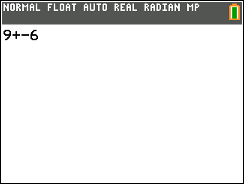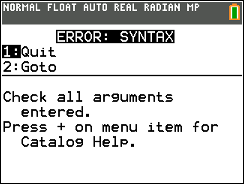Instead, to receive the expected result of 3 in this example, enter  [+] [(-)] .

Likewise, using the negative symbol [(-)] in place of the minus sign [-] will cause unexpected results:

TI-84 Plus CE

On the TI-84 Plus CE calculator, if you input a negative sign [(-)] between two numbers instead of the minus sign [-], the calculator will assume you intend to multiply the two numbers, and the result will be the product of the two values, as in this example: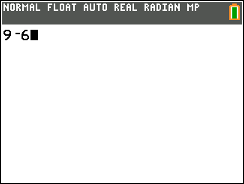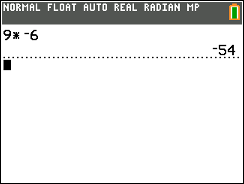Please Note: In TI-84 Plus CE Operating System version 5.7 and newer, the TI-84 Plus CE inserts the multiplication symbol [*] once the expression is evaluated. In OS versions 5.0 to 5.6, the implied multiplication is carried out, but no symbol is inserted.

TI-83 Plus, TI-84 Plus, and TI-84 Plus C Silver Edition

On the TI-83 Plus, TI-84 Plus, and TI-84 Plus C Silver Edition, using the negative [(-)] symbol in place of the minus sign [-] causes a Syntax Error to be generated.